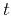Time-to-depth conversion and seismic velocity estimation using time-migration velocityNext: Partial differential equations for Up: Cameron, Fomel, Sethian: Velocity Previous: Time Migration Velocity

# Seismic Velocity

In this section, we will establish theoretical relationships between time-migration velocity and seismic velocity in 2-D and 3-D.

The seismic velocity and the Dix velocity are connected through the quantity, the geometrical spreading of image rays.is a scalar in 2-D and amatrix in 3-D. The simplest way to introduceis the following. Trace an image ray.is the starting surface point,is the traveltime. Call this ray central. Consider a small tube of rays around it. All these rays start from a small neighborhoodof the pointperpendicular to the earth surface. Thus, they represent a fragment of a plane wave propagating downward. Consider the fragment of the wave front defined by this ray tube at time. Letbe the fragment of the tangent to the front at the pointreached by the central ray at time, bounded by the ray tube (Figure 1). Then, in 2-D,is the derivative. In 3-D,is the matrix of the derivatives,, where derivatives are taken along certain mutually orthogonal directions,(Popov, 2002; Popov and Pšencik, 1978; Cervený, 2001).Qdef
Figure 1.
Illustration for the definition of geometrical spreading.The time evolution of the matricesandis given by(9)

whereit the velocity at the central ray at time,, andis theidentity matrix. The absolute value ofhas a simple meaning: it is the geometrical spreading of the image rays (Popov, 2002; Popov and Pšencik, 1978; Cervený, 2001). The matrix, introduced in the previous section, relates toandas. Hence,.

In (Cameron et al., 2007), we have proven that(10)

in 2-D, whereis the time-migration velocity, and(11)

in 3-D,is defined by equation 6 and can be determined from equation 7.Time-to-depth conversion and seismic velocity estimation using time-migration velocityNext: Partial differential equations for Up: Cameron, Fomel, Sethian: Velocity Previous: Time Migration Velocity

2013-03-02